Скачать презентацию Motion SWBAT define speed and acceleration relate acceleration

• Количество слайдов: 8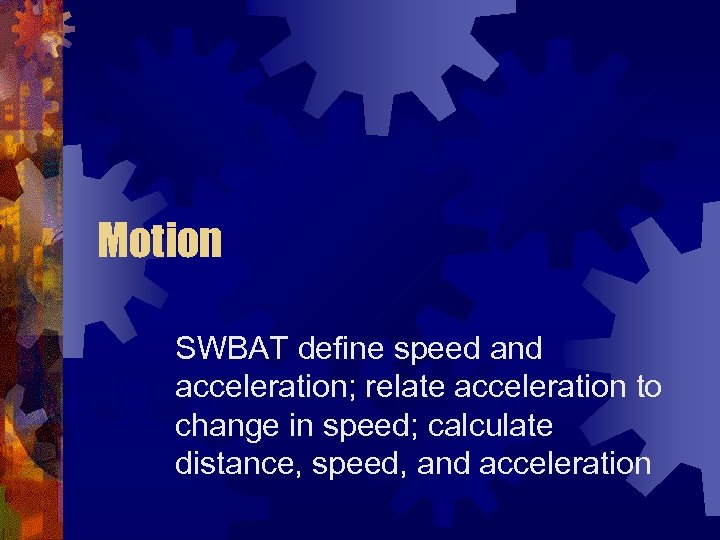Motion SWBAT define speed and acceleration; relate acceleration to change in speed; calculate distance, speed, and acceleration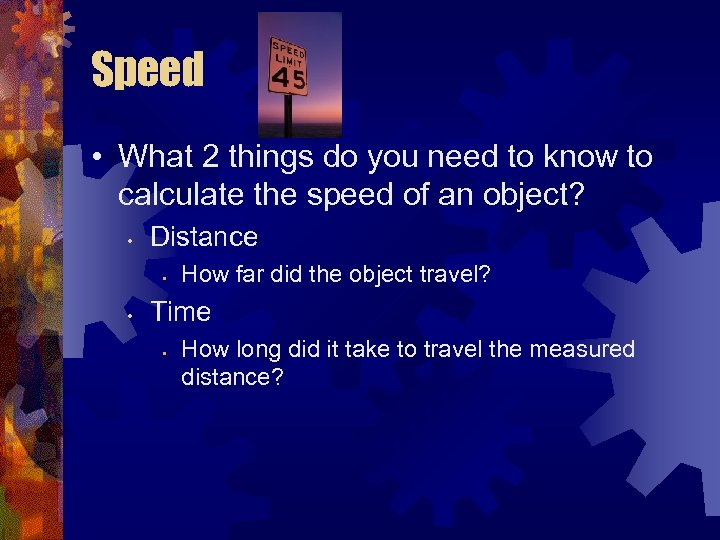Speed • What 2 things do you need to know to calculate the speed of an object? • Distance • • How far did the object travel? Time • How long did it take to travel the measured distance?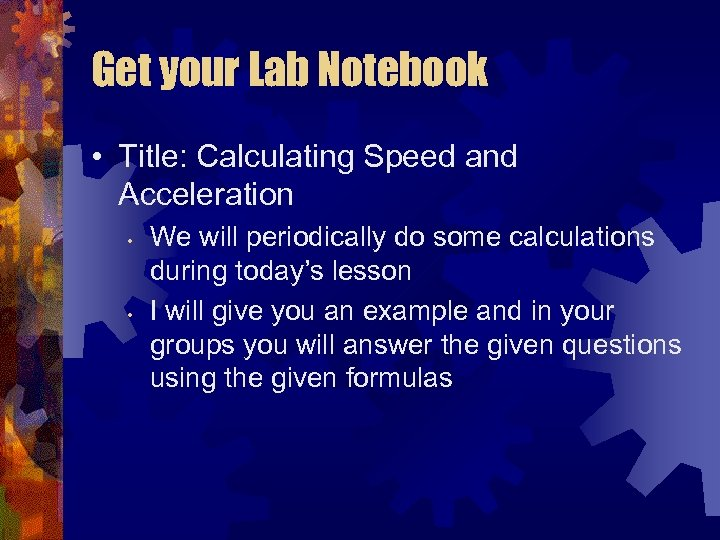Get your Lab Notebook • Title: Calculating Speed and Acceleration • • We will periodically do some calculations during today’s lesson I will give you an example and in your groups you will answer the given questions using the given formulas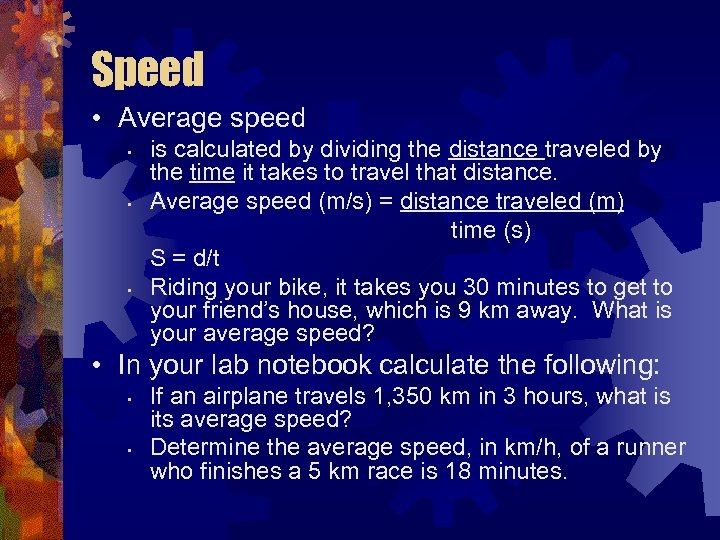Speed • Average speed • • • is calculated by dividing the distance traveled by the time it takes to travel that distance. Average speed (m/s) = distance traveled (m) time (s) S = d/t Riding your bike, it takes you 30 minutes to get to your friend’s house, which is 9 km away. What is your average speed? • In your lab notebook calculate the following: • • If an airplane travels 1, 350 km in 3 hours, what is its average speed? Determine the average speed, in km/h, of a runner who finishes a 5 km race is 18 minutes.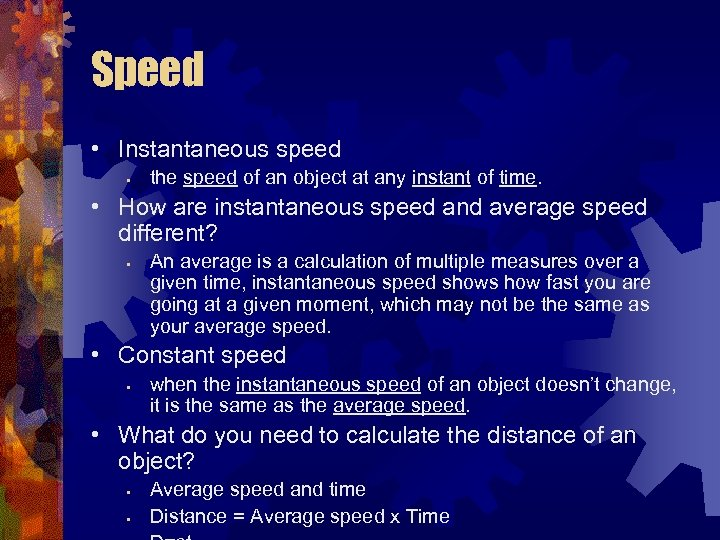Speed • Instantaneous speed • the speed of an object at any instant of time. • How are instantaneous speed and average speed different? • An average is a calculation of multiple measures over a given time, instantaneous speed shows how fast you are going at a given moment, which may not be the same as your average speed. • Constant speed • when the instantaneous speed of an object doesn’t change, it is the same as the average speed. • What do you need to calculate the distance of an object? • • Average speed and time Distance = Average speed x Time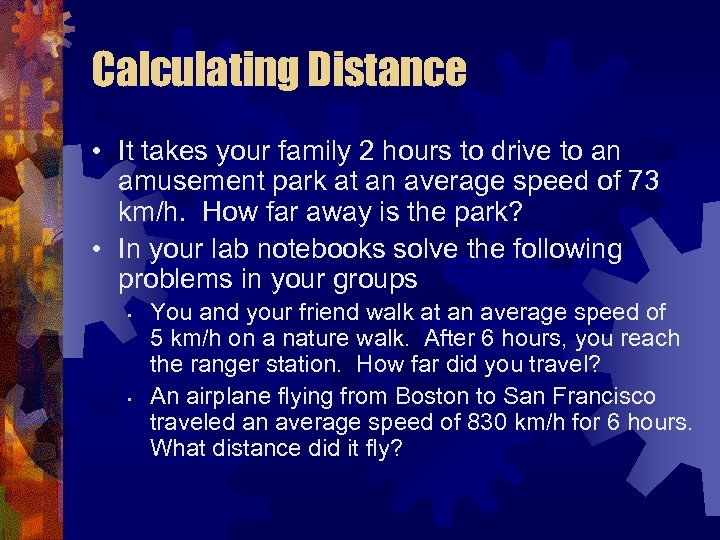Calculating Distance • It takes your family 2 hours to drive to an amusement park at an average speed of 73 km/h. How far away is the park? • In your lab notebooks solve the following problems in your groups • • You and your friend walk at an average speed of 5 km/h on a nature walk. After 6 hours, you reach the ranger station. How far did you travel? An airplane flying from Boston to San Francisco traveled an average speed of 830 km/h for 6 hours. What distance did it fly?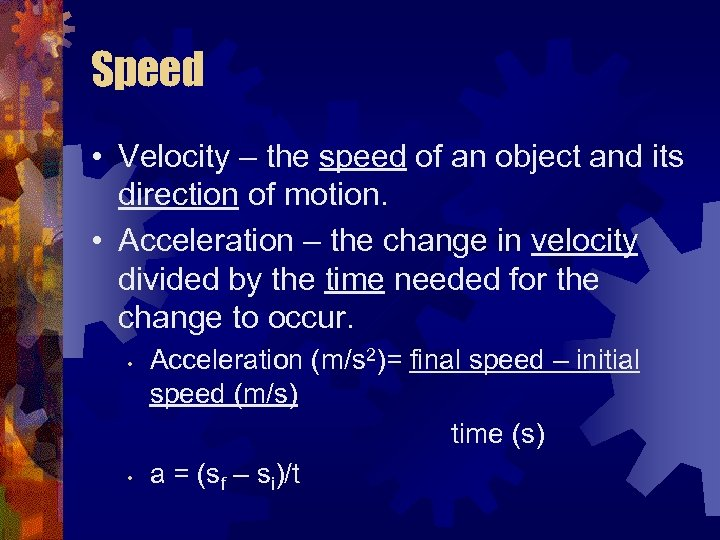Speed • Velocity – the speed of an object and its direction of motion. • Acceleration – the change in velocity divided by the time needed for the change to occur. • • Acceleration (m/s 2)= final speed – initial speed (m/s) time (s) a = (sf – si)/t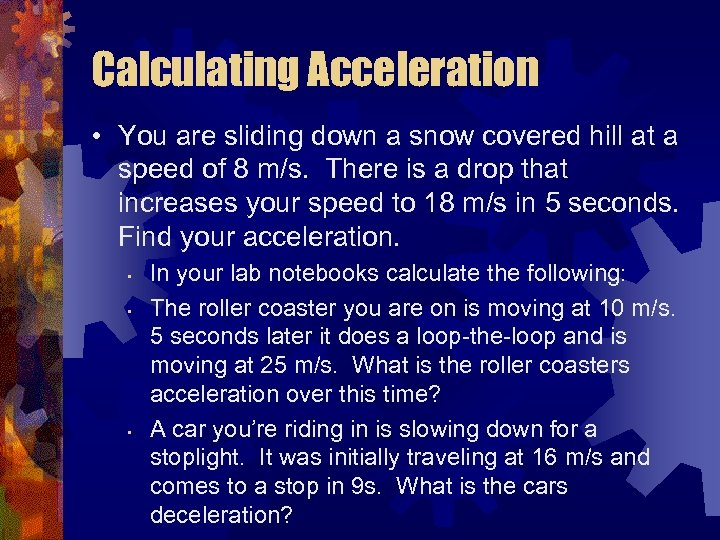Calculating Acceleration • You are sliding down a snow covered hill at a speed of 8 m/s. There is a drop that increases your speed to 18 m/s in 5 seconds. Find your acceleration. • • • In your lab notebooks calculate the following: The roller coaster you are on is moving at 10 m/s. 5 seconds later it does a loop-the-loop and is moving at 25 m/s. What is the roller coasters acceleration over this time? A car you’re riding in is slowing down for a stoplight. It was initially traveling at 16 m/s and comes to a stop in 9 s. What is the cars deceleration?